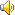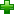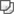assumed [ə'sju:md]英英解释

1. accepted as real or true without proof
2. taken as your right without justification
3. adopted in order to deceive

网络释义

assumed
假定的; 计算的; 假设的,计算的,理论的; 被假定;
assumed datum
假设起算值; 假定基准;
assumed median
假定中数; 假定中值;
assumed average
假定平均数; 假定平均数,假定平均值;
assumed interest
假定利息，设定利息; 假定利息;